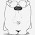## 2020-05-30

### 【醫生爸爸數學課】平方根#### 中小數學錦囊

1. 看清楚題目；
2. 寫數字，字要工整，位要對齊，很容易抄錯；
3. 數學基本功很重要，由加減乘除開始，一層一層加上去，如果下層根基不紮實，就像在沙上建房子，水一沖，隨即倒塌了 [註五 路加福音6:48-49]。
4. 抓緊定理，一理通，馬國明 ... 百理明。

#### 參考資料

[註三] 陳沛然 [醫生爸爸數學課] 分數、小數點和百分比 2019-02-01

2020年5月

#### 5 則留言:

1.2.多謝分享。不過，醫生爸爸似乎不太了解/高估小學數學課程了。（先吃飯，遲些有空再點評。）

3.陳醫生，謝謝你分享教孩子的tips！很有效！😊💪🏻

4.my solution,
sqrt(20)/5 + 3 * sqrt(1/5)
=sqrt(square(sqrt(20)/5)) + 3 * sqrt(1/5)
=sqrt(20/25) + 3 * sqrt(1/5)
=sqrt(4/5) + 3 * sqrt(1/5)
=sqrt(4)/sqrt(5) + 3 sqrt(1) / sqrt(5)
=2/sqrt(5) + 3 / sqrt(5)
= 5 / sqrt(5)
= sqrt(5)

5.fyi
https://www.mathcha.io/editor/y6MW8ilQtExUBrVWkPswgmZErfYBEQkjIk1l5E